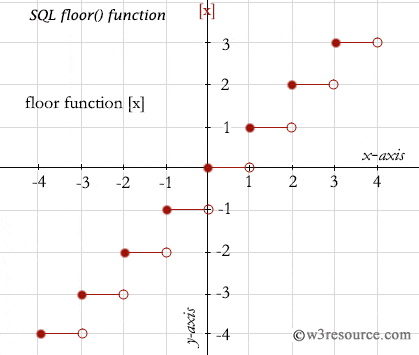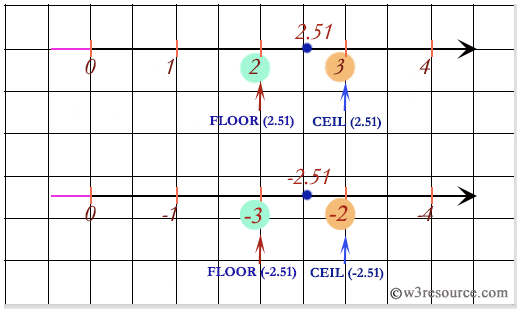# SQL FLOOR() function

## FLOOR() function

The SQL FLOOR() function rounded up any positive or negative decimal value down to the next least integer value. SQL DISTINCT along with the SQL FLOOR() function is used to retrieve only unique value after rounded down to the next least integer value depending on the column specified.

Syntax:

` FLOOR(expression) `

Parameters:

Name Description
expression An expression which is a numeric value or numeric data type. The bit data type is not allowed.

MySQL, PostgreSQL, SQL Server, and Oracle

All of above platforms support the SQL syntax of FLOOR().

## Pictorial presentation of FLOOR() FunctionExample:

To get the rounded down to next integer value of 17.36 from the DUAL table, the following SQL statement can be used:

``````SELECT FLOOR(17.36)
FROM dual;
``````

Output:

```FLOOR(17.36)
------------
17
```

## SQL FLOOR() function on negative value

To get the rounded down to next integer value of -17.36 from the DUAL table, the following SQL statement can be used :

``````SELECT FLOOR(-17.36)
FROM dual;
``````

Output:

```FLOOR(-17.36)
-------------
-18
```

## SQL FLOOR() function with distinct

To get the rounded down to next integer value of column 'commission' after multiplying by (-50) from the 'agents' table, the following SQL statement can be used :

``````SELECT DISTINCT(FLOOR(commission*(-50))) "DISTINCT(FLOOR())",
commission*(-50)
FROM agents;
``````

Output:

```DISTINCT(FLOOR()) COMMISSION*(-50)
----------------- ----------------
-6             -5.5
-8             -7.5
-6               -6
-7               -7
-7             -6.5
```

## SQL: Comparing between FLOOR() and CEIL() functionNote: Outputs of the said SQL statement shown here is taken by using Oracle Database 10g Express Edition.

Here is a slide presentation which covers the SQL arithmetic functions.

Practice SQL Exercises